This tour explores the use of gradient descent method for unconstrained and constrained optimization of a smooth function

Important: Please read the installation page for details about how to install the toolboxes. $\newcommand{\dotp}{\langle #1, #2 \rangle}$ $\newcommand{\pd}{ \frac{ \partial #1}{\partial #2} }$ $\newcommand{\umin}{\underset{#1}{\min}\;}$ $\newcommand{\qandq}{\quad\text{and}\quad}$ $\newcommand{\qwhereq}{\quad\text{where}\quad}$ $\newcommand{\qifq}{ \quad \text{if} \quad }$ $\newcommand{\ZZ}{\mathbb{Z}}$ $\newcommand{\RR}{\mathbb{R}}$ $\newcommand{\pa}{\left(#1\right)}$ $\newcommand{\si}{\sigma}$ $\newcommand{\Nn}{\mathcal{N}}$ $\newcommand{\Hh}{\mathcal{H}}$ $\newcommand{\Bb}{\mathcal{B}}$ $\newcommand{\EE}{\mathbb{E}}$ $\newcommand{\norm}{\|#1\|}$ $\newcommand{\abs}{\left|#1\right|}$ $\newcommand{\choice}{ \left\{ \begin{array}{l} #1 \end{array} \right. }$ $\newcommand{\al}{\alpha}$ $\newcommand{\la}{\lambda}$ $\newcommand{\ga}{\gamma}$ $\newcommand{\Ga}{\Gamma}$ $\newcommand{\La}{\Lambda}$ $\newcommand{\si}{\sigma}$ $\newcommand{\Si}{\Sigma}$ $\newcommand{\be}{\beta}$ $\newcommand{\de}{\delta}$ $\newcommand{\De}{\Delta}$ $\newcommand{\phi}{\varphi}$ $\newcommand{\th}{\theta}$ $\newcommand{\om}{\omega}$ $\newcommand{\Om}{\Omega}$

In :
using PyPlot
using NtToolBox
#arequire("NtToolBox")


## Gradient Descent for Unconstrained Problems¶

We consider the problem of finding a minimum of a function $f$, hence solving $$\umin{x \in \RR^d} f(x)$$ where $f : \RR^d \rightarrow \RR$ is a smooth function.

Note that the minimum is not necessarily unique. In the general case, $f$ might exhibit local minima, in which case the proposed algorithms is not expected to find a global minimizer of the problem. In this tour, we restrict our attention to convex function, so that the methods will converge to a global minimizer.

The simplest method is the gradient descent, that computes $$x^{(k+1)} = x^{(k)} - \tau_k \nabla f(x^{(k)}),$$ where $\tau_k>0$ is a step size, and $\nabla f(x) \in \RR^d$ is the gradient of $f$ at the point $x$, and $x^{(0)} \in \RR^d$ is any initial point.

In the convex case, if $f$ is of class $C^2$, in order to ensure convergence, the step size should satisfy $$0 < \tau_k < \frac{2}{ \sup_x \norm{Hf(x)} }$$ where $Hf(x) \in \RR^{d \times d}$ is the Hessian of $f$ at $x$ and $\norm{\cdot}$ is the spectral operator norm (largest eigenvalue).

We consider a simple problem, corresponding to the minimization of a 2-D quadratic form $$f(x) = \frac{1}{2} \pa{ x_1^2 + \eta x_2^2 } ,$$ where $\eta>0$ controls the anisotropy, and hence the difficulty, of the problem.

Anisotropy parameter $\eta$.

In :
eta = 10;


Function $f$.

In :
f = x -> ( x[1,1]^2 + eta*x[2, 1]^2 ) / 2;


Background image of the function.

In :
include("NtToolBox/src/ndgrid.jl")
t = linspace(-.7,.7,101)
(u, v) = meshgrid(t,t)
F = ( u .^ 2 + eta .* v .^ 2 ) ./ 2;


Display the function as a 2-D image.

In :
contourf(t, t, F, 35)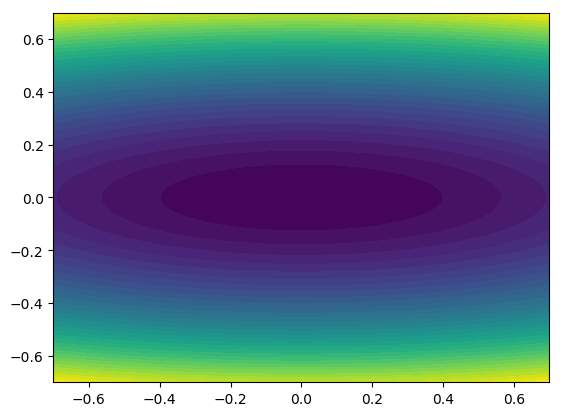Out:
PyObject <matplotlib.contour.QuadContourSet object at 0x0000000000DABAC8>

In :
GradF = x -> [[x[1, 1]],[eta.*x[2, 1]]];


The step size should satisfy $\tau_k < 2/\eta$. We use here a constant step size.

In :
tau = 1.8/eta;


Exercice 1: Perform the gradient descent using a fixed step size $\tau_k=\tau$. Display the decay of the energy $f(x^{(k)})$ through the iteration. Save the iterates so that |X(:,k)| corresponds to $x^{(k)}$.

In :
## Insert your code here.


Display the iterations.

In :
#contourf(t,t,Jmesh,35)
#plot(X[0,:], X[1,:], 'k.-')


Display the iteration for several different step sizes.

In :
## Insert your code here.


## Gradient and Divergence of Images¶

Local differential operators like gradient, divergence and laplacian are the building blocks for variational image processing.

Load an image $x_0 \in \RR^N$ of $N=n \times n$ pixels.

In :
n = 256
name = "NtToolBox/src/data/lena.png"


Display it.

In :
imageplot(x0)For a continuous function $g$, the gradient reads $$\nabla g(s) = \pa{ \pd{g(s)}{s_1}, \pd{g(s)}{s_2} } \in \RR^2.$$ (note that here, the variable $d$ denotes the 2-D spacial position).

We discretize this differential operator on a discrete image $x \in \RR^N$ using first order finite differences. $$(\nabla x)_i = ( x_{i_1,i_2}-x_{i_1-1,i_2}, x_{i_1,i_2}-x_{i_1,i_2-1} ) \in \RR^2.$$ Note that for simplity we use periodic boundary conditions.

Compute its gradient, using finite differences.

In :
grad = x -> cat(3, x - [x[end, :]'; x[1:end-1, :]], x - [x[:, end] x[:,1:end-1]]);


One thus has $\nabla : \RR^N \mapsto \RR^{N \times 2}.$

In :
v = grad(x0);


One can display each of its components.

In :
imageplot(v[:,:,1], L"\frac{d}{dx}", (1,2,1))
imageplot(v[:,:,2], L"\frac{d}{dy}", (1,2,2))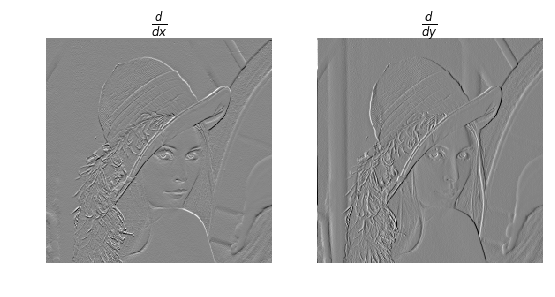Out:
PyObject <matplotlib.text.Text object at 0x0000000000E92240>

One can display its magnitude $\norm{(\nabla x)_i}$, which is large near edges.

In :
imageplot(sqrt(sum(v .* v, 3))[:, :])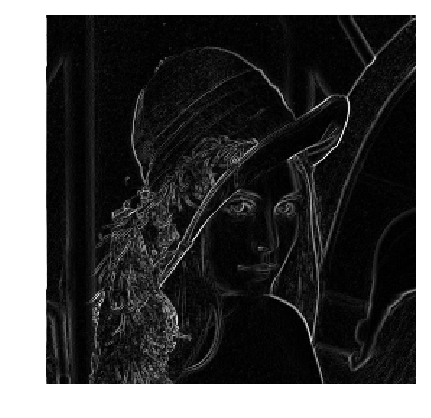The divergence operator maps vector field to images. For continuous vector fields (v(s) \in \RR^2), it is defined as $$\text{div}(v)(s) = \pd{v_1(s)}{s_1} + \pd{v_2(s)}{s_2} \in \RR.$$ (note that here, the variable (s) denotes the 2-D spacial position). It is minus the adjoint of the gadient, i.e. $\text{div} = - \nabla^*$.

It is discretized, for $v=(v^1,v^2)$ as $$\text{div}(v)_i = v^1_{i_1+1,i_2} - v^1_{i_1,i_2} + v^2_{i_1,i_2+1} - v^2_{i_1,i_2} .$$

In :
diiv = v -> [v[2:end, :, 1]; v[1, :, 1]'] - v[:, :, 1] + [v[:, 2:end, 2] v[:, 1, 2]] - v[:, :, 2]; # Pour ne pas avoir conflit avec la fonction div de Julia.

Out:
(::#9) (generic function with 1 method)

The Laplacian operatore is defined as $\Delta=\text{div} \circ \nabla = -\nabla^* \circ \nabla$. It is thus a negative symmetric operator.

In :
delta = x -> diiv(grad(x));


Display $\Delta x_0$.

In :
imageplot(delta(x0))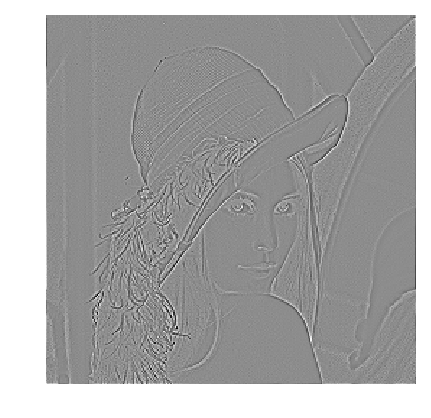Check that the relation $\norm{\nabla x} = - \dotp{\Delta x}{x}.$

In :
print("Should be 0:\n", sum(grad(x0) .* grad(x0)) + sum(delta(x0) .* x0) )

Should be 0:
0.0

## Gradient Descent in Image Processing¶

We consider now the problem of denoising an image $y \in \RR^d$ where $d = n \times n$ is the number of pixels ($n$ being the number of rows/columns in the image).

Add noise to the original image, to simulate a noisy image.

In :
sigma = 0.1
y = x0 + sigma .* randn(n,n);


Display the noisy image $y$.

In :
imageplot(clamP(y))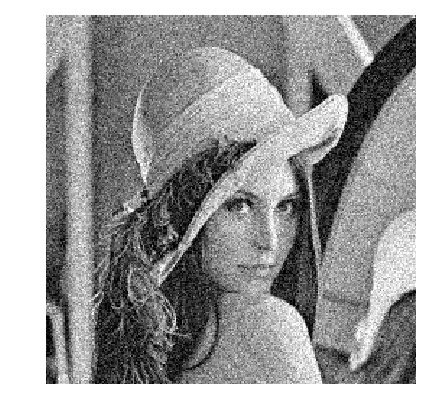Denoising is obtained by minimizing the following functional $$\umin{x \in \RR^d} f(x) = \frac{1}{2} \norm{y-x}^2 + \la J_\epsilon(x)$$ where $J_\epsilon(x)$ is a smoothed total variation of the image. $$J_\epsilon(x) = \sum_i \norm{ (G x)_i }_{\epsilon}$$ where $(Gx)_i \in \RR^2$ is an approximation of the gradient of $x$ at pixel $i$ and for $u \in \RR^2$, we use the following smoothing of the $L^2$ norm in $\RR^2$ $$\norm{u}_\epsilon = \sqrt{ \epsilon^2 + \norm{u}^2 },$$ for a small value of $\epsilon>0$.

The gradient of the functional read $$\nabla f(x) = x-y + \lambda \nabla J_\epsilon(x)$$ where the gradient of the smoothed TV norm is $$\nabla J_\epsilon(x)_i = G^*( u ) \qwhereq u_i = \frac{ (G x)_i }{\norm{ (G x)_i }_\epsilon}$$ where $G^*$ is the adjoint operator of $G$ which corresponds to minus a discretized divergence

Value for $\lambda$.

In :
la = 0.3 / 5;


Value for $\epsilon$.

In :
epsilon = 1e-3;


TV norm.

In :
norm_eps = u -> sqrt(eps^2 + sum(u .* u, 3))


Function $f$ to minimize.

In :
f = x -> 1/2 ^ norm(x - y)^2 + la .* J(x);


Gradient of $J_\epsilon$. Note that |div| implement $-G^*$.

In :
normalize_vec = (u, epsilon) -> u ./ repeat(reshape(NormEps(u,epsilon), (1, 1, 2)), inner = [size(NormEps(u,epsilon)), size(NormEps(u,epsilon)), 1])


In :
Gradf = x -> x - y + la .* gradTV(x);


The step size should satisfy $$0 < \tau_k < \frac{2}{ 1 + 4 \lambda / \epsilon }.$$ Here we use a slightly larger step size, which still work in practice.

In :
tau = 1.8/( 1 + la*8/epsilon )
tau = tau*4;


Exercice 3: Implement the gradient descent. Monitor the decay of $f$ through the iterations.

In :
## Insert your code here.


Display the resulting denoised image.

In :
#imageplot(clamp(x))


## Constrained Optimization Using Projected Gradient Descent¶

We consider a linear imaging operator $\Phi : x \mapsto \Phi(x)$ that maps high resolution images to low dimensional observations. Here we consider a pixel masking operator, that is diagonal over the spacial domain.

To emphasis the effect of the TV functional, we use a simple geometric image.

In :
n = 64
t = linspace(-1,1,n)
(Y,X) = meshgrid(t,t)
x0 = (max( abs(X), abs(Y) ) .< radius) .* 1.0;


We consider here the inpainting problem. This simply corresponds to a masking operator. Here we remove the central part of the image.

In :
a = 4
Lambda = ones(n, n)
Lambda[Int(n/2) - a : Int(n/2) + a, :] = 0;


Masking operator $\Phi$. Note that it is symmetric, i.e. $\Phi^*=\Phi$.

In :
Phi  = x -> x .* Lambda
PhiS = Phi;


Noiseless observations $y=\Phi x_0$.

In :
y = Phi(x0);


Display.

In :
imageplot(x0, "Original", (1,2,1))
imageplot(y, "Damaged", (1,2,2))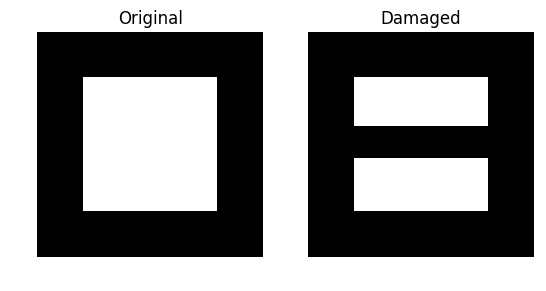Out:
PyObject <matplotlib.text.Text object at 0x0000000001351400>

We want to solve the noiseless inverse problem $y=\Phi f$ using a total variation regularization: $$\umin{ y=\Phi x } J_\epsilon(x).$$ We use the following projected gradient descent $$x^{(k+1)} = \text{Proj}_{\Hh}( x^{(k)} - \tau_k \nabla J_{\epsilon}(x^{(k)}) )$$ where $\text{Proj}_{\Hh}$ is the orthogonal projection on the set of linear constraint $\Phi x = y$, and is easy to compute for inpainting

In :
ProjH = x -> x + PhiS( y - Phi(x) );


Exercice 4: Display the evolution of the inpainting process.

In :
## Insert your code here.


Exercice 5: Try with several values of $\epsilon$.

In :
## Insert your code here.